# CMOS Logic Gates

## Digital Circuits

• #### Question 1

Counting practice: count from zero to thirty-one in binary, octal, and hexadecimal:• #### Question 2

 Don’t just sit there! Build something!!

Learning to analyze digital circuits requires much study and practice. Typically, students practice by working through lots of sample problems and checking their answers against those provided by the textbook or the instructor. While this is good, there is a much better way.

You will learn much more by actually building and analyzing real circuits, letting your test equipment provide the “answers” instead of a book or another person. For successful circuit-building exercises, follow these steps:

1. Draw the schematic diagram for the digital circuit to be analyzed.
2. Carefully build this circuit on a breadboard or other convenient medium.
3. Check the accuracy of the circuit’s construction, following each wire to each connection point, and verifying these elements one-by-one on the diagram.
4. Analyze the circuit, determining all output logic states for given input conditions.
5. Carefully measure those logic states, to verify the accuracy of your analysis.
6. If there are any errors, carefully check your circuit’s construction against the diagram, then carefully re-analyze the circuit and re-measure.

Always be sure that the power supply voltage levels are within specification for the logic circuits you plan to use. If TTL, the power supply must be a 5-volt regulated supply, adjusted to a value as close to 5.0 volts DC as possible.

One way you can save time and reduce the possibility of error is to begin with a very simple circuit and incrementally add components to increase its complexity after each analysis, rather than building a whole new circuit for each practice problem. Another time-saving technique is to re-use the same components in a variety of different circuit configurations. This way, you won’t have to measure any component’s value more than once.

• #### Question 3

Identify each of these logic gates by name, and complete their respective truth tables:• #### Question 4

The simplest type of digital logic circuit is an inverter, also called an inverting buffer, or NOT gate. Here is a schematic diagram for an inverter gate constructed from complementary MOSFETs (CMOS), shown connected to a SPDT switch and an LED:Determine the status of the LED in each of the input switch’s two positions. Denote the logic level of switch and LED in the form of a truth table: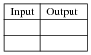• #### Question 5

Practical CMOS logic gates contain more than just MOSFETs. Here is a schematic diagram for a typical inverter gate circuit, with protection diodes:Explain what specific conditions each protection diode protects against.

• #### Question 6

A student builds the following digital circuit on a solderless breadboard (a “proto-board”):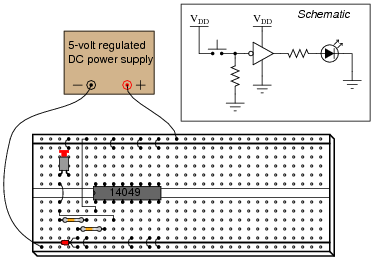The DIP circuit is a hex inverter (it contains six “inverter” or “NOT” logic gates), but only one of these gates is being used in this circuit. The student’s intent was to build a logic circuit that energized the LED when the pushbutton switch was unactuated, and de-energized the LED when the switch was pressed: so that the LED indicates the reverse state of the switch itself. The student builds this circuit, and it is found to function perfectly well.

Explain the purpose of the resistor on the input of the inverter. What is it there for? What might happen if it were to be removed from the circuit?

Also, explain why the inputs of all the unused inverter gates in this IC have been either connected to ground or to VDD. Is this necessary for the circuit to work properly, or is it just a precautionary measure?

• #### Question 7

The following is an internal schematic of a CMOS logic gate. Based on your analysis of the transistor circuit, determine what type of gate (AND, OR, NAND, NOR, XOR, etc.) it is:• #### Question 8

What is the typical power supply voltage range for a CD4xxx series (or MC4xxx series) CMOS logic gate? How does this compare with the allowable power supply voltage range for a standard (5 volt) TTL logic gate? Consult a datasheet for your answer.

• #### Question 9

A logic probe is a very useful tool for working with digital logic circuits. It indicates “high” and “low” logic states by means of LED’s, giving visual indication only if the voltage levels are appropriate for each state.

Here is a schematic diagram for a logic probe built using comparators. Each comparator has a threshold adjustment potentiometer, so that it may be set to indicate its respective logic state only if the signal voltage is well within the range stated by the logic manufacturer: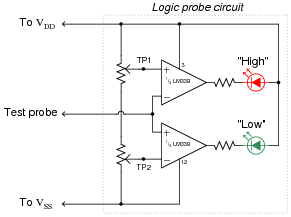When this logic probe circuit is connected to the VDD and VSS power supply terminals of a powered CMOS circuit, what voltage levels should test points TP1 and TP2 be adjusted to, in order for the probe to properly indicate “high” and “low” CMOS logic states? Consult a datasheet for the quad NAND gate numbered 4011. This is a legacy CMOS integrated circuit.

• #### Question 10

Here is a schematic diagram for a simple electronic combination lock, controlling power to a door lock solenoid:The four pushbutton switches (a, b, c, and d) are accessible to the person wishing to enter the door. The four toggle switches (A, B, C, and D) are located behind the door, and are used to set the code necessary for entering.

Explain how this system is supposed to work. What are the logic states of the respective gate outputs when a matching code is entered through the pushbutton switches? How about when a non-matching code is entered?

Do you see any security problems with this door lock circuit? How easy would it be for someone to enter, who does not know the four-bit code? Do you have any suggestions for improving this lock design?

• #### Question 11

Many modern CMOS gate circuits are buffered with additional transistor stages on their outputs. For example, an unbuffered AND gate is shown here, with no more transistors than is necessary to fulfill the “AND” logic function:One type of “buffered” CMOS AND gate looks like this:As far as the basic logic function is concerned, the additional transistors are unnecessary. However, the “buffering” they provide does serve a useful function. What is that function? Are there any disadvantages to buffered logic gates, versus unbuffered?

• #### Question 12

In the early days of solid-state logic gate circuit technology, there was a very clear distinction between TTL and CMOS. TTL gates were capable of switching on and off very fast, required a tightly regulated power supply voltage, and used a lot of power. CMOS gates were not quite as fast as TTL, but could tolerate a much wider range of power supply voltages and were far less wasteful on power.

Then, during the 1980’s a new technology known as high-speed CMOS, or HCMOS, entered the scene. Explain what HCMOS is, how it compares to the older TTL and CMOS families (54/74xx and 4xxx number series, respectively), and where it is often used. Hint: high-speed CMOS bears the same numerical codes as the old TTL 54xx and 74xx series ICs (e.g. 74HC00 instead of 7400).

• #### Question 13

In high-speed digital circuits, a very important logic gate parameter is propagation delay: the delay time between a change-of-state on a gate’s input and the corresponding change-of-state on that gate’s output. Consult a manufacturer’s datasheet for any CMOS logic gate and report the typical propagation delay times published there.

Also, explain what causes propagation delay in logic gates. Why isn’t the change in output state instantaneous when an input changes states?

• #### Question 14

Logic gates are limited in the number of gate inputs which one output can reliably drive. This limit is referred to as fan-out:Explain why this limit exists. What is it about the construction of CMOS logic gates that inherently limits the number of CMOS inputs that any one CMOS output can drive? What might happen if this limit is exceeded?

Fan-out for CMOS is a quite different than fan-out for TTL. Most importantly is that CMOS fan-out is inversely proportional to operating frequency. Explain why.

• #### Question 15

An important parameter of logic gate circuitry is noise margin. What exactly is “noise margin,” and how is it defined for logic gates?

Specifically, how much noise margin do digital circuits exclusively composed of CMOS gates have? How does this compare with the noise margin of all-TTL circuitry?

Note: you will need to consult CMOS gate datasheets to answer this question properly.

• #### Question 16

A trend in CMOS logic gate development is toward lower and lower operating voltages. The “AUC” family of CMOS logic, for example, is able to operate at less than 2 volts VDD!

Explain why this is a trend in modern logic circuit design. What benefits result from lower operating voltages? What possible disadvantages also result?

• #### Question 17

Predict how the operation of this logic gate circuit will be affected as a result of the following faults. Consider each fault independently (i.e. one at a time, no multiple faults):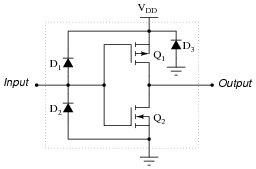Diode D1 fails open:
Diode D1 fails shorted:
Diode D2 fails open:
Diode D2 fails shorted:
Transistor Q1 fails open (drain to source):
Transistor Q2 fails open (drain to source):

For each of these conditions, explain why the resulting effects will occur.

• #### Question 18

Identify at least three different transistor faults in this CMOS logic gate that could cause the output to fail low:• #### Question 19

A student builds the following circuit to demonstrate the behavior of a NAND gate: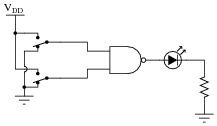When the student tests the circuit, though, something is wrong:

Both switches LOW, no light.
One switch HIGH, the other switch LOW; LED lights up.
One switch LOW, the other switch HIGH; LED lights up.
Both switches HIGH, no light.

Instead of acting as a NAND gate should, it seems to behave as if it were an Exclusive-OR gate! Examining the circuit for mistakes, the student discovers missing power connections to the chip - in other words, neither VDD nor VSS are connected to the power source.

While this certainly is a problem, the student is left to wonder, “How did the circuit ever function at all?” With no power connected to the chip, how is it possible that the LED ever lit in any condition?

• #### Question 20

What does it mean if you see a logic gate symbol in a schematic diagram with a strange-looking “S” figure drawn inside of it?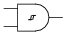• #### Question 21

In CMOS circuitry, one side of the DC power supply is usually labeled as “VDD”, while the other side is labeled as “VSS”. Why is this? What do the subscripts “DD” and “SS” represent?

• #### Question 22

A very important concept to understand in digital circuitry is the difference between current sourcing and current sinking. For instance, examine this CMOS inverting buffer gate circuit, connected to a load:Is this gate circuit configured to source load current, sink load current, or do both?

• #### Question 23

Suppose that a CMOS inverting buffer gate were to drive a predominantly inductive load, such as a small relay coil:Normally, it would be considered good design practice to connect a commutating diode in parallel with the relay coil, to prevent high-voltage transients when the coil is de-energized. However, this is not necessary when a CMOS gate drives a coil. Explain why.

• #### Question 24

A problem unique to certain types of CMOS logic gates is something called SCR latchup. This is an abnormal condition capable of ruining a circuit, or at the very least causing operational problems in a circuit. Explain what this phenomenon is, and what causes it.

• #### Question 25

What logic state does a floating CMOS gate input naturally assume? How does this compare against traditional TTL?

• #### Question 26

As an electronics instructor, I have the opportunity to see a lot of creative mistakes made by students as they learn to build circuits. One very common mistake made in CMOS circuit construction manifests itself in erratic behavior: the circuit may function correctly for a time, but suddenly and randomly it stops. Then, just by waving your hand next to the circuit, it begins to work again!

This problem is especially prevalent on days where the atmospheric humidity is low, and static electric charges easily accumulate on objects and people. Explain what sort of CMOS wiring mistake would cause a powered logic gate to behave erratically due to nearby static electric fields, and what the proper solution is to this problem.

• #### Question 27

Logic probes are useful tools for troubleshooting digital logic gate circuits, but they certainly have limitations. For instance, in this simple circuit, a logic probe will give correct “high” and “low” readings at test point 1 (TP1), but it will always read “low” (even when the LED is on) at test point 2 (TP2):Now, obviously the output of the gate is “high” when the LED is on, otherwise it would not receive enough voltage to illuminate. Why then does a logic probe fail to indicate a high logic state at TP2?